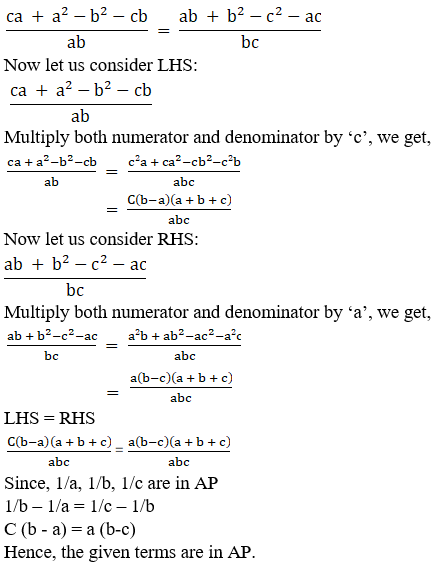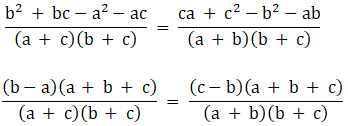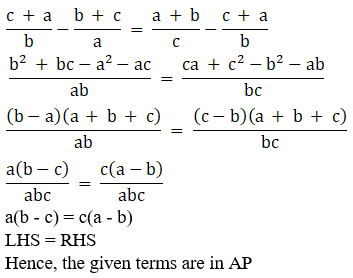• +91 9971497814
• info@interviewmaterial.com

# RD Chapter 19- Arithmetic Progressions Ex-19.5 Interview Questions Answers

### Related Subjects

Question 1 :

If 1/a, 1/b, 1/c are in A.P., prove that:

(i) (b+c)/a, (c+a)/b, (a+b)/c are in A.P.

(ii) a(b + c), b(c + a), c(a + b) are in A.P.

(i) (b+c)/a, (c+a)/b,(a+b)/c are in A.P.

We know that, if a, b,c are in AP, then b – a = c – b

If, 1/a, 1/b, 1/c arein AP

Then, 1/b – 1/a = 1/c– 1/b

If (b+c)/a, (c+a)/b,(a+b)/c are in AP

Then, (c+a)/b –(b+c)/a = (a+b)/c – (c+a)/b

Let us take LCMSince, 1/a, 1/b, 1/c arein AP

1/b – 1/a = 1/c – 1/b

C (b – a) = a (b-c)

Hence, the given termsare in AP.

(ii) a(b + c), b(c + a),c(a + b) are in A.P.

We know that if, b(c +a) – a(b+c) = c(a+b) – b(c+a)

Consider LHS:

b(c + a) – a(b+c)

Upon simplification weget,

b(c + a) – a(b+c) = bc+ ba – ab – ac

= c (b-a)

Now,

c(a+b) – b(c+a) = ca +cb – bc – ba

= a (c-b)

We know,

1/a, 1/b, 1/c are inAP

So, 1/a – 1/b = 1/b –1/c

Or c(b-a) = a(c-b)

Hence, given terms arein AP.

Question 2 :

If a2, b2, c2 are in AP., provethat a/(b+c), b/(c+a), c/(a+b) are in AP.

If a2, b2,c2 are in AP then, b2 – a2 = c2 –b2

If a/(b+c), b/(c+a),c/(a+b) are in AP then,

b/(c+a) – a/(b+c) =c/(a+b) – b/(c+a)

Let us take LCM onboth the sides we get,Since, b2 –a2 = c2 – b2

Substituting b2 –a2= c2 – b2 in above, we get

LHS = RHS

Hence, given terms arein AP

Question 3 :

If a, b, c are in A.P., then show that:
(i) a2(b + c), b2(c + a), c2(a + b) are alsoin A.P.
(ii) b + c – a, c + a – b, a + b – c are in A.P.
(iii) bc – a2, ca – b2, ab – c2 are inA.P.

(i) a2(b + c),b2(c + a), c2(a + b) are also in A.P.

If b2(c +a) – a2(b + c) = c2(a + b) – b2(c + a)

b2c + b2a– a2b – a2c = c2a + c2b – b2a– b2c

Given, b – a = c – b

And since a, b, c arein AP,

c(b2 –a2 ) + ab(b – a) = a(c2 – b2 )+ bc(c – b)

(b – a) (ab + bc + ca)= (c – b) (ab + bc + ca)

Upon cancelling, ab +bc + ca from both sides

b – a = c – b

2b = c + a [which istrue]

Hence, given terms arein AP

(ii) b + c – a, c + a– b, a + b – c are in A.P.

If (c + a – b) – (b +c – a) = (a + b – c) – (c + a – b)

Then, b + c – a, c + a– b, a + b – c are in A.P.

Let us consider LHSand RHS

(c + a – b) – (b + c –a) = (a + b – c) – (c + a – b)

2a – 2b = 2b – 2c

b – a = c – b

And since a, b, c arein AP,

b – a = c – b

Hence, given terms arein AP.

(iii) bc – a2,ca – b2, ab – c2 are in A.P.

If (ca – b2)– (bc – a2) = (ab – c2) – (ca – b2)

Then, bc – a2,ca – b2, ab – c2 are in A.P.

Let us consider LHSand RHS

(ca – b2) –(bc – a2) = (ab – c2) – (ca – b2)

(a – b2 –bc + a2) = (ab – c2 – ca + b2)

(a – b) (a + b + c) =(b – c) (a + b + c)

a – b = b – c

And since a, b, c arein AP,

b – c = a – b

Hence, given terms arein AP

Question 4 :

If (b+c)/a, (c+a)/b, (a+b)/c are in AP., prove that:

(i) 1/a, 1/b, 1/c are in AP

(ii) bc, ca, ab are in AP

(i) 1/a, 1/b, 1/c are in AP

If 1/a, 1/b, 1/c arein AP then,

1/b – 1/a = 1/c – 1/b

Let us consider LHS:

1/b – 1/a = (a-b)/ab

= c(a-b)/abc [bymultiplying with ‘c’ on both the numerator and denominator]

Let us consider RHS:

1/c – 1/b = (b-c)/bc

= a(b-c)/bc [bymultiplying with ‘a’ on both the numerator and denominator]

Since, (b+c)/a,(c+a)/b, (a+b)/c are in AP(ii) bc, ca, ab are in AP

If bc, ca, ab are inAP then,

ca – bc = ab – ca

c (a-b) = a (b-c)

If 1/a, 1/b, 1/c arein AP then,

1/b – 1/a = 1/c – 1/b

c (a-b) = a (b-c)

Hence, the given termsare in AP

Question 5 :

If a, b, c are in A.P., prove that:
(i) (a – c)2 = 4 (a – b) (b – c)

(ii) a2 + c2 + 4ac = 2 (ab + bc + ca)

(iii) a3 + c3 + 6abc = 8b3

(i) (a – c)2 =4 (a – b) (b – c)

Let us expand theabove expression

a2 + c2 –2ac = 4(ab – ac – b2 + bc)

a2 +4c2b2 + 2ac – 4ab – 4bc = 0

(a + c – 2b)2 =0

a + c – 2b = 0

Since a, b, c are inAP

b – a = c – b

a + c – 2b = 0

a + c = 2b

Hence, (a – c)2 =4 (a – b) (b – c)

(ii) a2 + c2 +4ac = 2 (ab + bc + ca)

Let us expand theabove expression

a2 + c2 +4ac = 2 (ab + bc + ca)

a2 + c2 +2ac – 2ab – 2bc = 0

(a + c – b)2 –b2 = 0

a + c – b = b

a + c – 2b = 0

2b = a+c

b = (a+c)/2

Since a, b, c are inAP

b – a = c – b

b = (a+c)/2

Hence, a2 +c2 + 4ac = 2 (ab + bc + ca)

(iii) a3 + c3 +6abc = 8b3

Let us expand theabove expression

a3 + c3 +6abc = 8b3

a3 + c3 –(2b)3 + 6abc = 0

a3 +(-2b)3 + c3 + 3a(-2b)c = 0

Since, if a + b + c =0, a3 + b3 + c3 = 3abc

(a – 2b + c)3 =0

a – 2b + c = 0

a + c = 2b

b = (a+c)/2

Since a, b, c are inAP

a – b = c – b

b = (a+c)/2

Hence, a3 +c3 + 6abc = 8b3

Question 6 :

If a(1/b + 1/c), b(1/c + 1/a), c(1/a + 1/b) are in AP., prove that a, b,c are in AP.

Here, we know a(1/b +1/c), b(1/c + 1/a), c(1/a + 1/b) are in AP

Also, a(1/b + 1/c) +1, b(1/c + 1/a) + 1, c(1/a + 1/b) + 1 are in AP

Let us take LCM foreach expression then we get,

(ac+ab+bc)/bc ,(ab+bc+ac)/ac, (cb+ac+ab)/ab are in AP

1/bc, 1/ac, 1/ab arein AP

Let us multiplynumerator with ‘abc’, we get

abc/bc, abc/ac, abc/abare in AP

a, b, c are in AP.

Hence proved.

Question 7 :

Show that x2 + xy + y2, z2 +zx + x2 and y2 + yz + z2 are inconsecutive terms of an A.P., if x, y and z are in A.P.

x, y, z are in AP

Given, x2 +xy + y2, z2 + zx + x2 and y2 +yz + z2 are in AP

(z2 +zx + x2) – (x2 + xy + y2) = (y2 +yz + z2) – (z2 + zx + x2)

Let d = commondifference,

So, Y = x + d and x =x + 2d

Let us consider theLHS:

(z2 +zx + x2) – (x2 + xy + y2)

z2 +zx – xy – y2

(x + 2d)2 +(x + 2d)x – x(x + d) – (x + d)2

x2 +4xd + 4d2 + x2 + 2xd – x2 – xd– x2 – 2xd – d2

3xd + 3d2

Now, let us considerRHS:

(y2 +yz + z2) – (z2 + zx + x2)

y2 +yz – zx – x2

(x + d)2 +(x + d)(x + 2d) – (x + 2d)x – x2

x2 +2dx + d2 + x2 + 2dx + xd + 2d2 –x2 – 2dx – x2

3xd + 3d2

LHS = RHS

x2 +xy + y2, z2 + zx + x2 and y2 +yz + z2 are in consecutive terms of A.P

Hence proved.

Todays Deals### RD Chapter 19- Arithmetic Progressions Ex-19.5 Contributorskrishan

Name:
Email:

# Latest News# 9000 interview questions in different categories